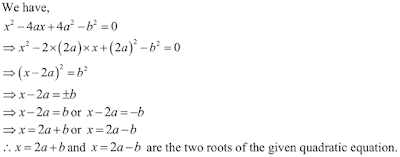## Chapter 8 Quadratic Equations R.D. Sharma Solutions for Class 10th Math Exercise 8.4

Find the roots of the following quadratic equations (if they exist) by the method of completing the square.

1. x2 - 4√2x + 6 = 0

Solution2. 2x2 - 7x + 3 = 0

Solution3. 3x2 + 11x + 10 = 0

Solution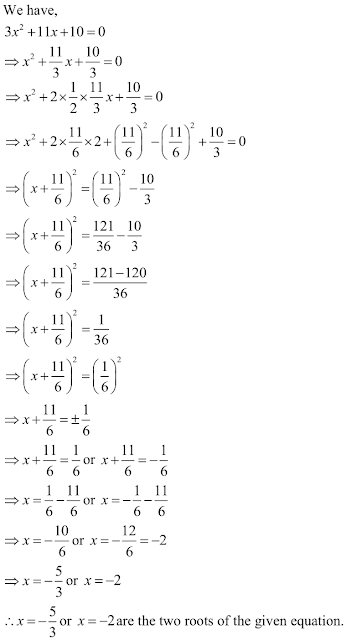4. 2x2 + x - 4 = 0

Solution5. 2x2 + x + 4 = 0

Solution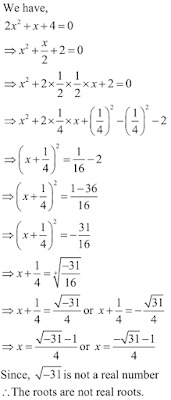6. 4x2 + 4√3 x + 3 = 0

Solution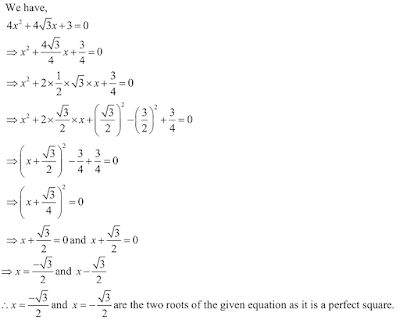7. √2 x2 -3 x - 2√2 = 0

Solution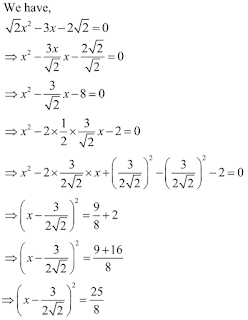8. √3 x2 + 10 x + 7√3 = 0

Solution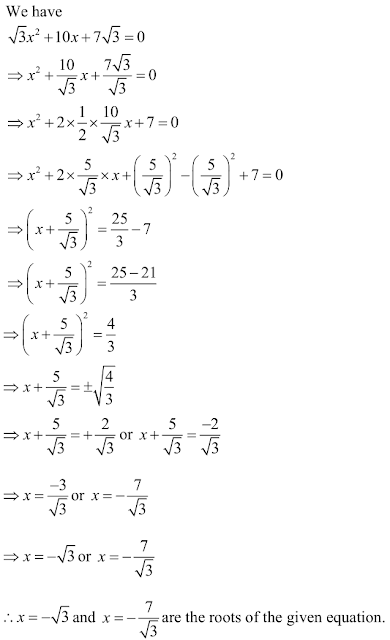9. x2 - (√2 + 1) x + √2 = 0

Solution10. x2 - 4ax + 4a2 = 0

Solution